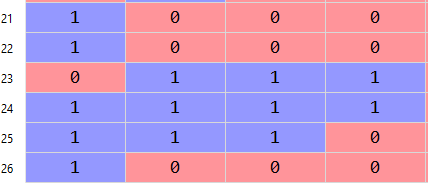# Multi-target classification problem:**Error** multi-target not supported for CrossEntropyLoss

I have a dataset which has multiple targets attributes.

Sample targets for 12 data points (4 attributes as target outputs: not one-hot encoded):`````` class Classifier(nn.Module):
def __init__(self,input_nodes):
super(Classifier, self).__init__()
self.fc3 = nn.Linear(75000, 500)
self.fc4 = nn.Linear(500, 200)
self.fc5 = nn.Linear(200, 100)
self.fc_out = nn.Linear(100, 4)
self.dropout = nn.Dropout(0.5)

def forward(self, x):
x = F.relu(self.fc3(x))
x = self.dropout(x)
x = F.relu(self.fc4(x))
x = self.dropout(x)
x = F.relu(self.fc5(x))
x = self.dropout(x)
x = self.fc_out(x)
return x

criterion = nn.CrossEntropyLoss()

for epoch in range(n_epochs):
running_loss = 0
i = 0
model.train()
y_hat  = model(data) # Shape of y_hat = 6*4 (as 4 targets)
loss = criterion(y_hat, label) # Shape of label = 6*4
#--> Give error: RuntimeError: multi-target not supported at ..\aten\src\THNN/generic/ClassNLLCriterion.c:20

loss.backward()
optimizer.step()
train_loss.append(loss.item())
train_losses.append(loss.item())
running_loss += loss.item()
``````

Error: RuntimeError: multi-target not supported at …\aten\src\THNN/generic/ClassNLLCriterion.c:20

How can I train and evaluate the multi-target classification problem?

Hello Animesh!

Just to confirm, are your really working with a “multi-target”
(multi-class) classification problem? I ask because the sample
targets you show in your .png image have either 0 (in just row 2)
or 1 (in the rest of the rows) of the labels set.

That is, even though you didn’t show such a row, could you have
a row (say, row 17) for which all four fields are set to 1?

If not, you have a multi-class (but not multi-label) classification
problem, and you should recast it as such, and (most likely)
use `nn.CrossEntropyLoss` as your loss function.

The general consensus is that MultiLabelSoftMarginLoss
problem.

Best.

K. Frank

Hi @KFrank,

That is, even though you didn’t show such a row, could you have
a row (say, row 17) for which all four fields are set to 1?

Yes, I have some rows with all 1s and some rows with more than ones there.

The targets can be like this:Hi Animesh,

I think you’re looking for BCEloss which can be found here.

Hi Prerna and Animesh!

You don’t want – in the typical case – `BCELoss` for classification
problems. This is because `BCELoss` requires the predictions fed
into it to be numbers in (0, 1) (probability-like numbers).

For example, Animesh’s output layer is a `Linear`

``````        self.fc_out = nn.Linear(100, 4)
``````

that will, in general, output numbers ranging from -infinity to
+infinity. You also don’t want to pass these outputs through
a `Sigmoid` (or `Softmax`) layer to map them to (0, 1) because
of the risk of overflow.

You want, instead, a loss function that takes logit-like
predictions (that run from -infinity to +infinity), such as
`BCEWithLogitsLoss`.

`MultiLabelSoftMarginLoss` and `BCEWithLogitsLoss`
are essentially the same function, as Peter explains here:

Good luck.

K. Frank

2 Likes